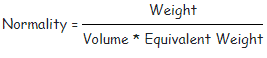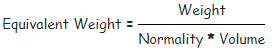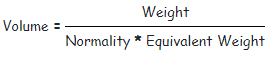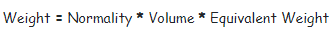Request a Tool

Neutralisation Calculator

A chemical reaction in which an acid and a base interact

Normality
0

Output

Equivalent weight
0

Output

Volume of Solvent
0

Weight of Solute
0

FormulaDefination / Uses

Neutralization refers to a chemical reaction in which an acid and a base interact to form water and salt as products.The method is based on the fact that one gram equivalent of the acid completely neutralizes one gram equivalent of the base and vice versa.

FormulaDefination / Uses

Neutralization refers to a chemical reaction in which an acid and a base interact to form water and salt as products.The method is based on the fact that one gram equivalent of the acid completely neutralizes one gram equivalent of the base and vice versa.

FormulaDefination / Uses

Neutralization refers to a chemical reaction in which an acid and a base interact to form water and salt as products.The method is based on the fact that one gram equivalent of the acid completely neutralizes one gram equivalent of the base and vice versa.

FormulaDefination / Uses

Neutralization refers to a chemical reaction in which an acid and a base interact to form water and salt as products.The method is based on the fact that one gram equivalent of the acid completely neutralizes one gram equivalent of the base and vice versa.

A neutralisation reaction is a chemical reaction that occurs when an acid and a base combine. The creation of a salt is the result of this process. The essential reaction in the case of strong acids and bases is the formation of water molecules by combining hydrogen ions with hydroxyl ions. The fact that 1g of base is entirely neutralised by 1g of acid supports the neutralisation reaction. The neutralisation calculator calculates the equivalent weight of an acid or base that will react with hydrogen or hydroxyl ions. To calculate equivalent weight, this calculator uses the normality of the solution, volume, and weight of acid or base in the solution. Use the upper given formula for manual calculations. No sign-up, registration OR captcha is required to use this tool.